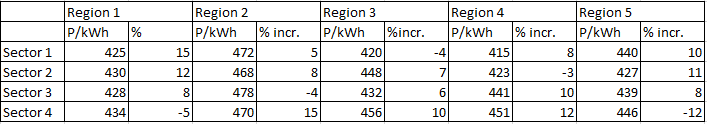### CAT 1997 Question Paper Question 22

Instructions

The following table gives the tariff [in paise per kilo-watt-hour (kWh)] levied by the UPSEB in 1994-95, in four sectors and the regions within them. The table also gives the percentage change in the tariff as compared to 1991-92.Question 22

# Which of the following statements is true?

Solution

Average tariff in region 4 = $$\frac{415 + 423 + 441+ 451}{4}$$ = $$\frac{1730}{4}$$ = 432.5 p/kWh => A is wrong.

In all the four sectors, tariff in region 2 is higher than tariff in region 5.

=> Average tariff in region 2 is higher than average tariff in region 5 => Option B is correct.

• All Quant CAT Formulas and shortcuts PDF
• 30+ CAT previous papers with solutions PDF